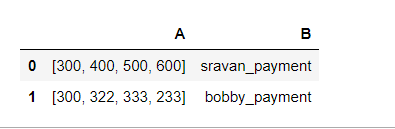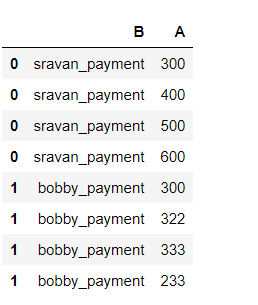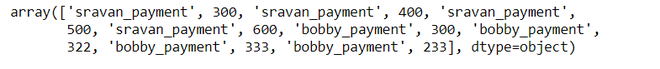# Flatten a list of DataFrames

In this article, we are going to see how to flatten a list of DataFrames. Flattening is defined as Converting or Changing data format to a narrow format. The advantage of the flattened list is Increases the computing speed and Good understanding of data.

Example:

Let consider, the data frame that contains values like payments in four months. Actually, the data is stored in a list format.Note: 0,1,2 are the indices of the records

Flattening means assigning lists separately for each author.We are going to perform flatten operations on the list using data frames.

Method 1:

Step 1: Create a simple data frame.

## Python3

 `#importing pandas module ` `import` `pandas as pd ` ` `  `#creating dataframe with 2 columns ` `df ``=` `pd.DataFrame(data``=``[[[ ``300``, ``400``, ``500``, ``600``], ``'sravan_payment'``],  ` `                        ``[[ ``300``, ``322``, ``333``, ``233``], ``'bobby_payment'``]],  ` `                  ``index``=``[ ``0``, ``1``], columns``=``[ ``'A'``, ``'B'``]) ` ` `  `display(df) `

Output:Step 2: iterate each row with a specific column.

## Python3

 `flatdata ``=` `pd.DataFrame([( index, value) ``for` `( index, values) ` `                         ``in` `df[ ``'A'` `].iteritems() ``for` `value ``in` `values], ` `                             ``columns ``=` `[ ``'index'``, ``'A'``]).set_index( ``'index'` `) ` ` `  `df ``=` `df.drop( ``'A'``, axis ``=` `1` `).join( flatdata ) ` `display(df)`

Output:Methods 2: Using the flatten methods.

We are going to apply the flatten function for the above code.

## Python3

 `#importing pandas module for dataframe. ` `import` `pandas as pd ` ` `  `df ``=` `pd.DataFrame(data``=``[[[ ``300``, ``400``, ``500``, ``600``], ``'sravan_payment'``],  ` `                        ``[[ ``300``, ``322``, ``333``, ``233``], ``'bobby_payment'``]],  ` `                  ``index ``=` `[ ``0``, ``1``], columns ``=` `[ ``'A'``, ``'B'``]) ` `display(df)`

Output:## Python3

 `df.values.flatten()`

Output:Whether you're preparing for your first job interview or aiming to upskill in this ever-evolving tech landscape, GeeksforGeeks Courses are your key to success. We provide top-quality content at affordable prices, all geared towards accelerating your growth in a time-bound manner. Join the millions we've already empowered, and we're here to do the same for you. Don't miss out - check it out now!

Previous
Next